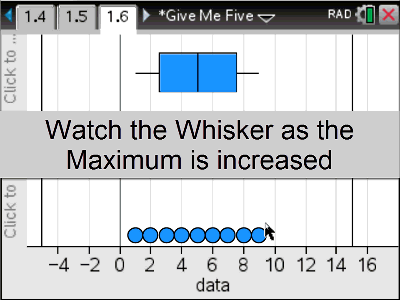# Activities

•• ##### Author60 Minutes

4.4

## Give Me Five#### Activity Overview

This activity explores the calculations of the five figure summary: {min, Q1, median, Q3 and max}. How is an outlier determined? What is positive / negative skew? By physically dragging the maximum point students can determine when the whisker ‘breaks’ and an outlier is created. The connection between the dot plot and the box and whisker plot improves the visual power of this dynamic interaction.

#### Objectives

To describe the centre and spread of a univariate data set by way of a 5-figure summary and visually by a box & whisker plot.

#### Vocabulary

 Minimum Q1 = Quartile 1 Median ( Q2 ) Q3 = Quartile 3 Maximum Outlier Upper Fence Lower Fence Left / Right Skew Box and Whisker Plot